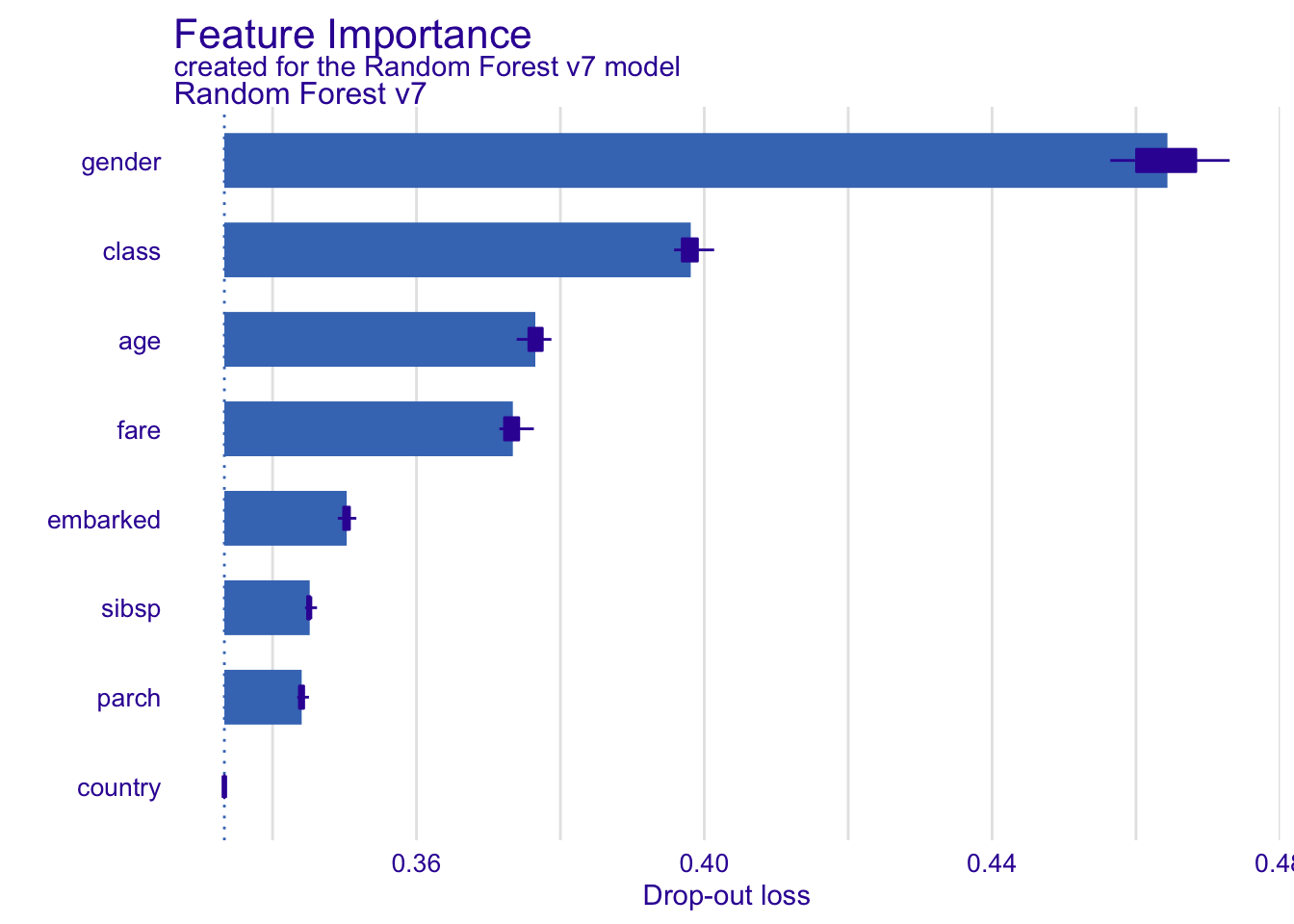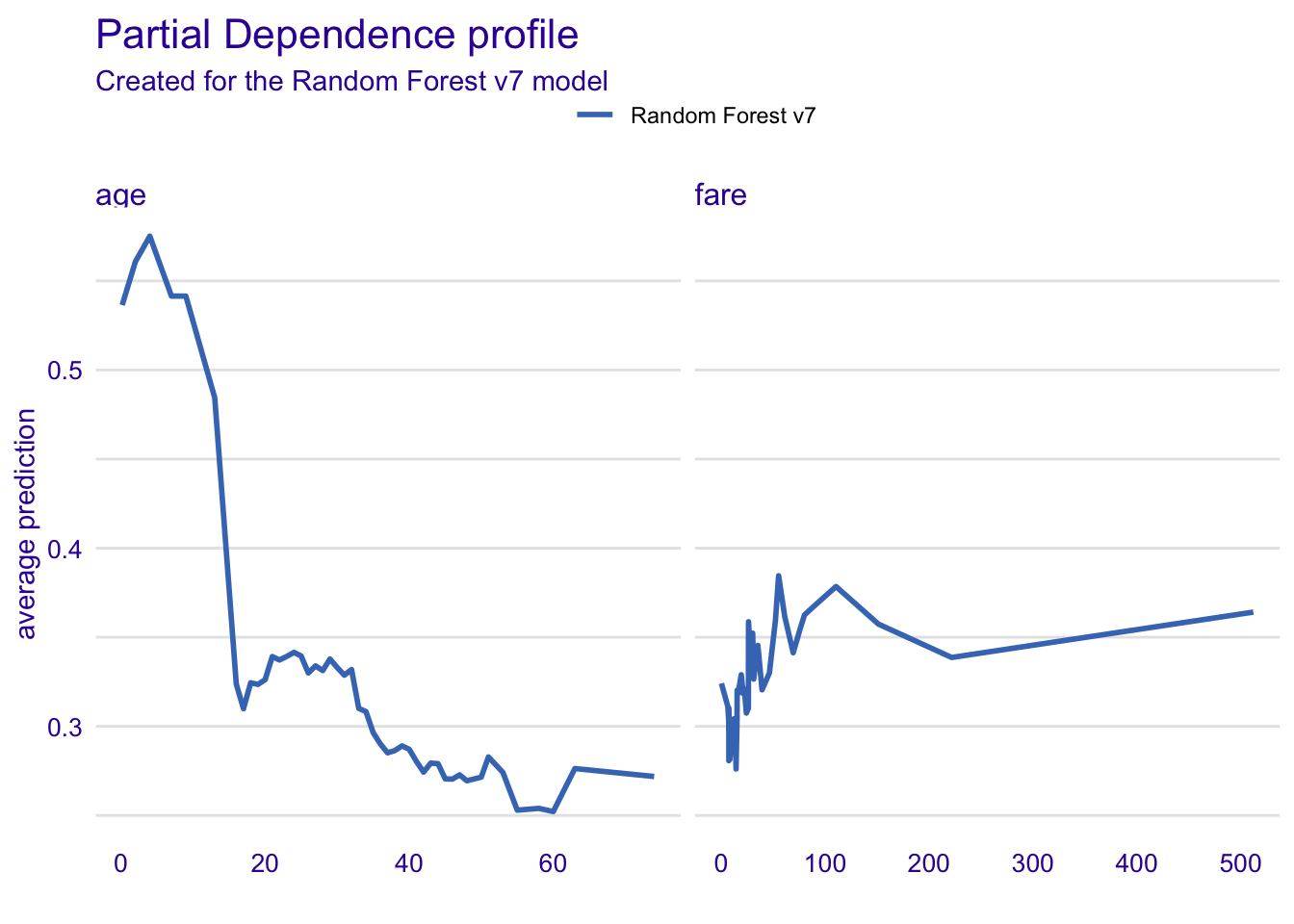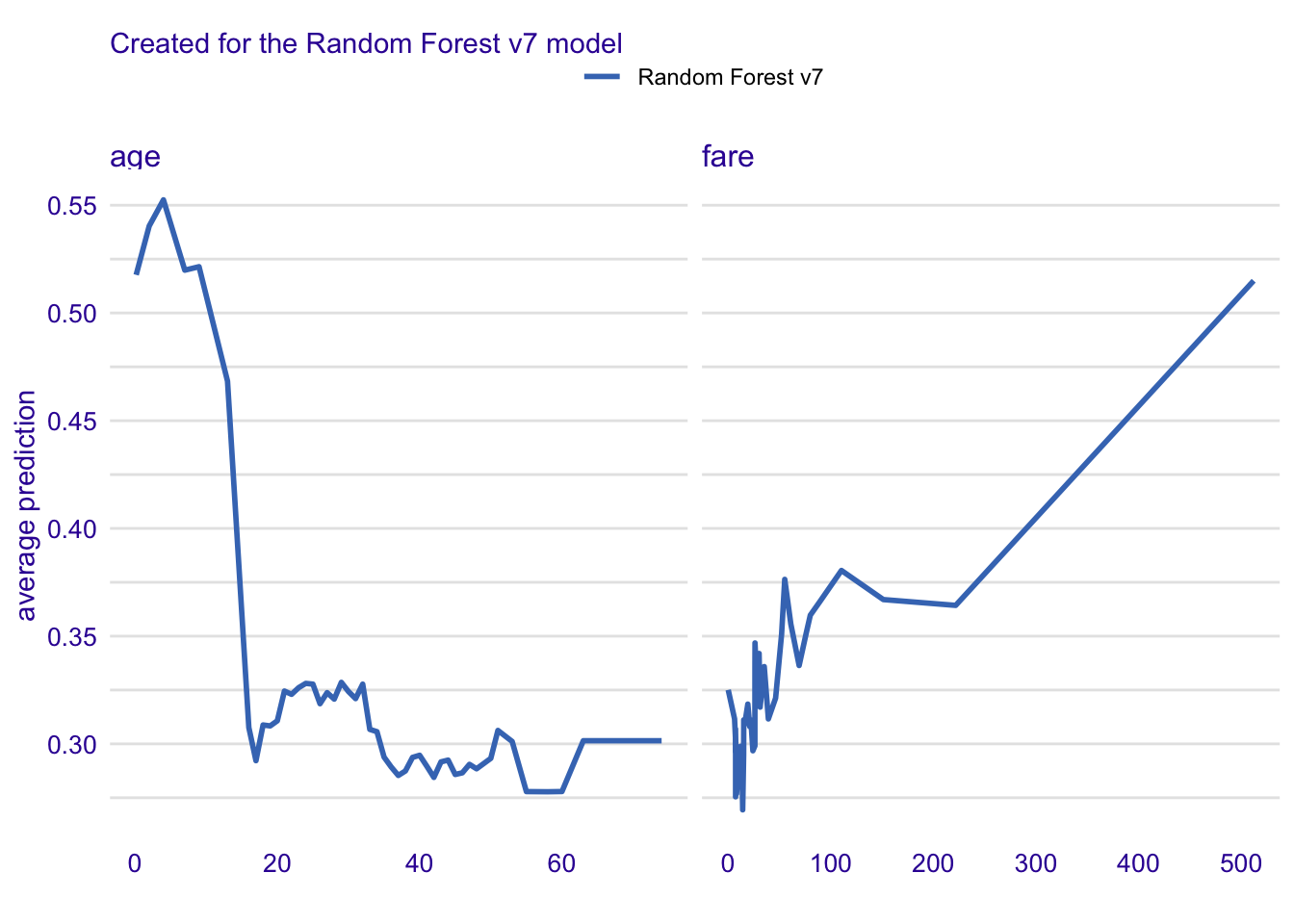# Data for Titanic survival

Let’s see an example for `DALEX` package for classification models for the survival problem for Titanic dataset. Here we are using a dataset `titanic` avaliable in the `DALEX` package. Note that this data was copied from the `stablelearner` package.

``````library("DALEX")
``````#>   gender age class    embarked       country  fare sibsp parch survived
#> 1   male  42   3rd Southampton United States  7.11     0     0       no
#> 2   male  13   3rd Southampton United States 20.05     0     2       no
#> 3   male  16   3rd Southampton United States 20.05     1     1       no
#> 4 female  39   3rd Southampton       England 20.05     1     1      yes
#> 5 female  16   3rd Southampton        Norway  7.13     0     0      yes
#> 6   male  25   3rd Southampton United States  7.13     0     0      yes``````

# Model for Titanic survival

Ok, now it’s time to create a model. Let’s use the Random Forest model.

``````# prepare model
library("randomForest")
titanic <- na.omit(titanic)
model_titanic_rf <- randomForest(survived == "yes" ~ gender + age + class + embarked +
fare + sibsp + parch,  data = titanic)
model_titanic_rf``````
``````#>
#> Call:
#>  randomForest(formula = survived == "yes" ~ gender + age + class +      embarked + fare + sibsp + parch, data = titanic)
#>                Type of random forest: regression
#>                      Number of trees: 500
#> No. of variables tried at each split: 2
#>
#>           Mean of squared residuals: 0.143236
#>                     % Var explained: 34.65``````

# Explainer for Titanic survival

The third step (it’s optional but useful) is to create a `DALEX` explainer for random forest model.

``````library("DALEX")
explain_titanic_rf <- explain(model_titanic_rf,
data = titanic[,-9],
y = titanic\$survived == "yes",
label = "Random Forest v7")``````
``````#> Preparation of a new explainer is initiated
#>   -> model label       :  Random Forest v7
#>   -> data              :  2099  rows  8  cols
#>   -> target variable   :  2099  values
#>   -> model_info        :  package randomForest , ver. 4.6.14 , task regression ( [33m default [39m )
#>   -> predict function  :  yhat.randomForest  will be used ( [33m default [39m )
#>   -> predicted values  :  numerical, min =  0.01286123 , mean =  0.3248356 , max =  0.9912115
#>   -> residual function :  difference between y and yhat ( [33m default [39m )
#>   -> residuals         :  numerical, min =  -0.779851 , mean =  -0.0003954087 , max =  0.9085878
#>  [32m A new explainer has been created! [39m``````

# Model Level Feature Importance

Use the `feature_importance()` explainer to present importance of particular features. Note that `type = "difference"` normalizes dropouts, and now they all start in 0.

``````library("ingredients")

fi_rf <- feature_importance(explain_titanic_rf)
``````#>       variable mean_dropout_loss            label
#> 1 _full_model_         0.3332983 Random Forest v7
#> 2      country         0.3332983 Random Forest v7
#> 3        parch         0.3440449 Random Forest v7
#> 4        sibsp         0.3451616 Random Forest v7
#> 5     embarked         0.3503033 Random Forest v7
#> 6         fare         0.3733943 Random Forest v7``````
``plot(fi_rf)``# Feature effects

As we see the most important feature is `gender`. Next three importnat features are `class`, `age` and `fare`. Let’s see the link between model response and these features.

Such univariate relation can be calculated with `partial_dependence()`.

## age

Kids 5 years old and younger have much higher survival probability.

### Partial Dependence Profiles

``````pp_age  <- partial_dependence(explain_titanic_rf, variables =  c("age", "fare"))
``````#> Top profiles    :
#>   _vname_          _label_       _x_    _yhat_ _ids_
#> 1    fare Random Forest v7 0.0000000 0.3241036     0
#> 2     age Random Forest v7 0.1666667 0.5364253     0
#> 3     age Random Forest v7 2.0000000 0.5607931     0
#> 4     age Random Forest v7 4.0000000 0.5750886     0
#> 5    fare Random Forest v7 6.1904000 0.3111265     0
#> 6     age Random Forest v7 7.0000000 0.5414633     0``````
``plot(pp_age)``### Conditional Dependence Profiles

``````cp_age  <- conditional_dependence(explain_titanic_rf, variables =  c("age", "fare"))
plot(cp_age)``````### Accumulated Local Effect Profiles

``````ap_age  <- accumulated_dependence(explain_titanic_rf, variables =  c("age", "fare"))
plot(ap_age)``````